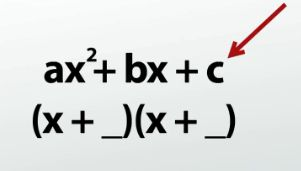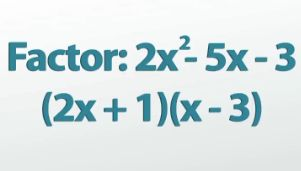# Factoring Quadratic Equations: Polynomial Problems with a Non-1 Leading Coefficient

An error occurred trying to load this video.

Try refreshing the page, or contact customer support.

Coming up next: How to Complete the Square

### You're on a roll. Keep up the good work!

Replay
Your next lesson will play in 10 seconds
• 0:04 Factoring Quadratic Equations
• 1:34 Adjusting The Pattern
• 5:03 Another Example
• 6:47 Lesson Summary

Want to watch this again later?

Timeline
Autoplay
Autoplay
Speed

#### Recommended Lessons and Courses for You

Lesson Transcript
Instructor: Luke Winspur

Luke has taught high school algebra and geometry, college calculus, and has a master's degree in education.

Once you get good at factoring quadratics with 1x squared in the front of the expression, it's time to try ones with numbers other than 1. It will be the same general idea, but there are a few extra steps to learn. Do that here!

## Factoring Quadratic EquationsThis lesson will be on advanced factoring techniques, so I'm going to assume that you know a few things: first, that factoring is the process of breaking up a number into the things that we can multiply together to get that number. This means that factoring a quadratic expression is the process of taking a trinomial and turning it into multiplication of two binomials - basically FOIL backwards. We do this by looking for a pair of numbers that have a product equal to the constant on the end of the trinomial and a sum equal to the coefficient on the x's. For example, turning x2 - 3x - 10 into (x + 2)(x - 5) by realizing that 2 * -5 = -10, and 2 + -5 = -3.

So, where do we go from here? What else is there to know? Well, the method I just described only works for quadratic trinomials where the leading coefficient (the number in front of the x squared) is equal to 1. As soon as we're asked to factor something else, like 2x2 - 5x - 3, we're going to be in trouble. The goal is still the same - split the trinomial into a product of binomials - and we'll still find a lot of the same patterns, but now we'll have to make two slight changes in the process in order to end up with the correct answer. Let's go ahead and take a look at the example I just mentioned.

## Adjusting the Pattern

Factor 2x2 - 5x - 3.We'll start this problem very similarly to the simple factoring problems by looking for two numbers that fit the pattern. The thing is, it's not going to be quite the same pattern. One part stays the same, and that is the fact that we need to find a pair of numbers that will add up to the middle coefficient - in this case, -5. But the second part is going to be different. Instead of our two numbers needing to have a product equal to the constant on the end, we now need the product of our pair of numbers to be equal to the constant on the end times the leading coefficient. This was actually true for the easier factoring problems as well, but the leading coefficient was just 1, so multiplying by 1 didn't change what the number was.

The next step of the problem should be familiar. Find a pair of numbers that has a sum of -5 and a product of -6. Making a quick list of the factor pairs of 6 and keeping in mind that we'll need one to be positive and one to be negative, makes it clear that -6 and +1 are our two winners. And now we come to the only other difference in our process. Instead of simply being able to say -6 and +1 go in our binomials and we're done, there is an extra step before we can be sure of our answer. While there are multiple ways of doing this step, I recommend using the area method to work your way backward to the answer. Putting the 2x2 and the -3 in one diagonal of the chart and the two values we came up with using our pattern attached with x's in the other diagonal, gets us one step away from our answer.

To unlock this lesson you must be a Study.com Member.

### Register to view this lesson

Are you a student or a teacher?

### Unlock Your Education

#### See for yourself why 30 million people use Study.com

##### Become a Study.com member and start learning now.
Back
What teachers are saying about Study.com

### Earning College Credit

Did you know… We have over 160 college courses that prepare you to earn credit by exam that is accepted by over 1,500 colleges and universities. You can test out of the first two years of college and save thousands off your degree. Anyone can earn credit-by-exam regardless of age or education level.